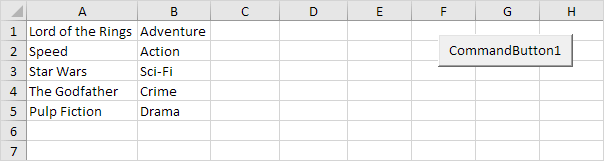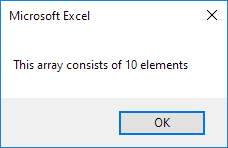# Size of an Array

To get the size of an array in Excel VBA, you can use the UBound and LBound functions.

Place a command button on your worksheet and add the following code lines:

1. First, we need to declare the array. Our array has two dimensions. It consists of 5 rows and 2 columns. Also declare two variables of type Integer.

Dim Films(1 To 5, 1 To 2) As String, x As Integer, y As Integer

The array may look like this.2. Next, we get the size of the array. Add the following code lines:

x = UBound(Films, 1) - LBound(Films, 1) + 1
y = UBound(Films, 2) - LBound(Films, 2) + 1

UBound(Films, 1) gives the upper limit of the first dimension, which is 5.
LBound(Films, 1) gives the lower limit of the first dimension, which is 1.

UBound(Films, 2) gives the upper limit of the second dimension, which is 2.
LBound(Films, 2) gives the lower limit of the second dimension, which is 1.

As a result, x equals 5 and y equals 2.

3. We use a MsgBox to display the number of elements of the array.

MsgBox "This array consists of " & x * y & " elements"

Result:Go to Next Chapter: Function and Sub